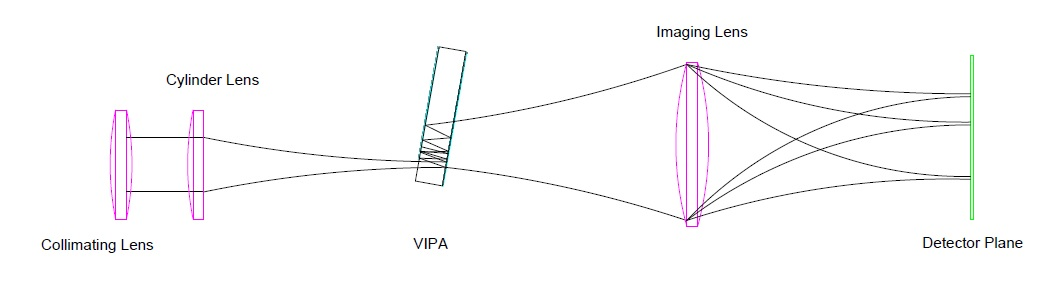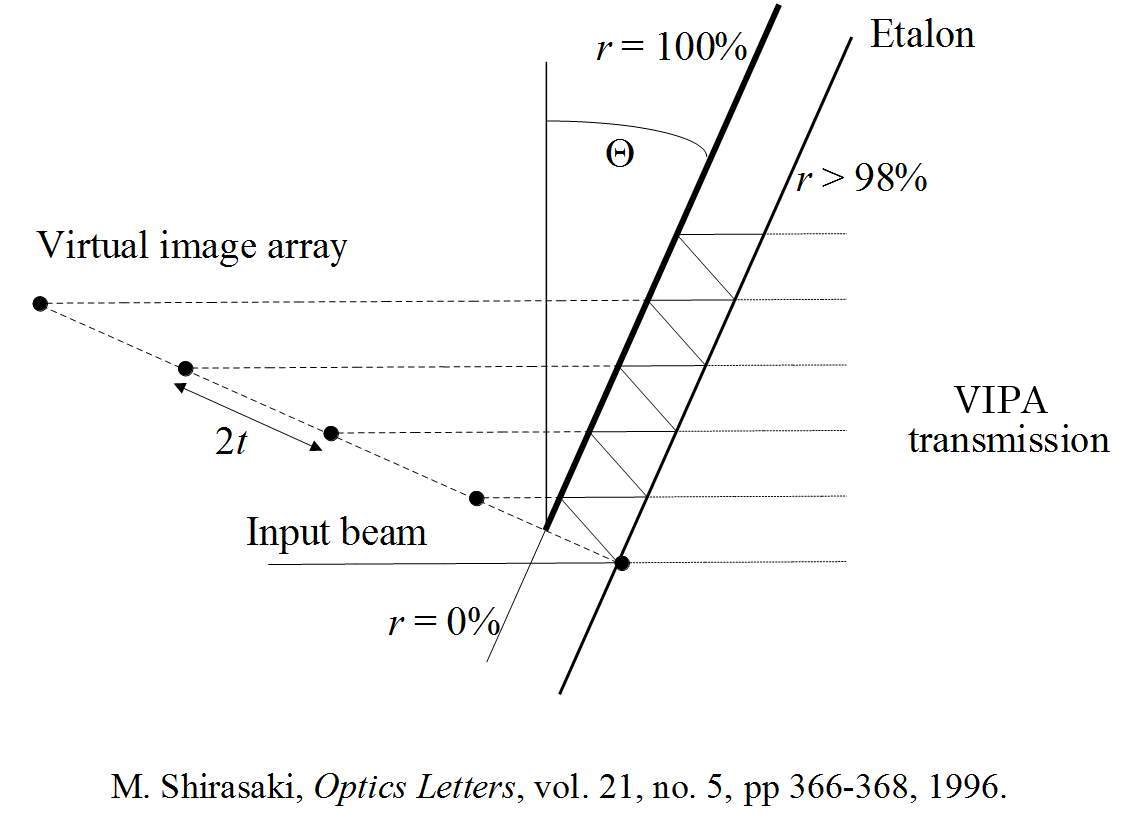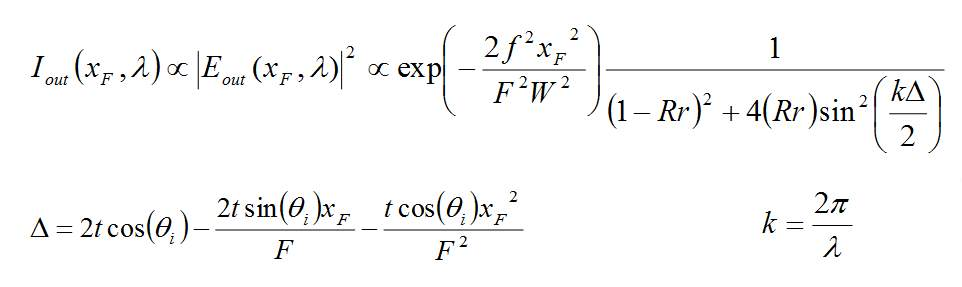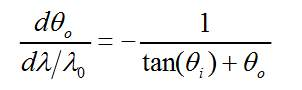#### Introduction

The VIPA is a relatively new interferometric device. It is based upon the principle of a side entrance etalon. The device is composed of two plane parallel mirrors with one a total reflector and the other a partial reflector. The incident angle and the single sided exit for the device ensure both high spectral dispersion and optical efficiency.

The LightMachinery VIPA calculator is a simple design tool for determining the performance of a VIPA etalon. The tool calculates VIPA performance using a paraxial solution1,2. Reference papers used in the development of this calculator can be found in the Bibliography at the end of this note.

#### VIPA Principles

The virtually imaged phase array (VIPA) is a high resolution, wavelength dispersion device. Initially finding application in wavelength division multiplexing/demultiplexing3 for telecommunications, VIPA’s high resolution, high dispersion properties have been utilized in optical fibre dispersion4 and pulsed laser chirp compensation5.

A VIPA is composed of two plane parallel mirrors, one a near total reflector and the other a partial reflector, Figure 1. The light source of interest is focused to a waist using a cylindrical lens. The input beam is typically input to the VIPA at one edge at near normal incidence. The near normal angle of incidence ensures that many reflections between the two surfaces occur along the VIPA’s length.Figure 1, Simple VIPA Layout

Optimum coupling of the input beam to the VIPA without clipping occurs when the waist of the input beam is placed on the surface of the partial reflector. The mode is then of equal size at the input to the VIPA and on its first bounce off the total reflector. A tightly focused waist and a small transition zone from transmission to total reflection on the first surface ensures that the VIPA incident angle is kept small. This increases the number of round trips per unit length of the VIPA and keeps the over device length small.

The optimum VIPA size is determined by the input mode, output surface reflectivity and incident angle. The input beam, such as from an optical fiber is first collimated, determining the VIPA width. A cylindrical lens is then used to form a tight line focus as noted above. With each round trip a small portion of the input light exits the VIPA. VIPA output surfaces are typically greater than 90% reflectors over the wavelength range of interest. The number of round trips is then determined by:

IR / IO = (R x r)N              where: IR / IO is the intensity fraction

R is the reflectivity of the high reflector

r is the reflectivity of the partial reflector

N is the number of bounces

By picking a fraction of the input intensity below which beam interference is ignored (typically 1%), the number of round trips can be calculated. Combined with the increasing mode diameter along the length of the VIPA, and its incident angle, the required length of the VIPA can be calculated.

Along the VIPA length, interference between the overlapping mode round trips produces a wavelength dispersing response. Each VIPA round trip appears as a series of displaced beams each having a fixed phase relationship.Figure 2, Virtual Image Phase Relationship

The transmission from the VIPA is then brought to a focus with an output lens. The lens resolves the spectral content of the input beam at the lens focal plane in a direction perpendicular to the input beam. The intensity function of the output is given by:where:         W  is the collimated input mode diameter

xF is the position along the focal plane x axis

λ is the input wavelength

F is the focal length of the output lens

f is the focal length of the input lens

Using the paraxial approximation, the wavelength dispersion of the VIPA can be rewritten in terms of an angular dispersion originating at the z position of the output focal lens:where:             θo is the output angle

θi is the incident angle

It is important to note that the dispersion is not a linear function but determined by angular position in the output plane.

#### Calculator Features

The VIPA calculator used by LightMachinery adds several helpful features beyond the basic VIPA theory outlined in the previous section:

Index of refraction – the model includes the VIPA index in its calculation allowing it to model both parallel mirrors in air and solid VIPAs.

X and Y cylindrical output lenses – the calculator deals with the output beam in the x and y planes independently. This allows the designer the flexibility to optimize the output beam size to match the detector used to monitor the spectra.

Manufacturing tolerances – several formulae determining the impact on etalon finesse of manufacturing tolerances have been included in the calculator. These values allow the designer to evaluate whether particular specifications impact the final device performance.

Material loss – a feature which is not yet implemented in the current version of software is the ability to calculate the impact of substrate losses on the performance of the VIPA.

Transition AR to HR – the calculator also includes the ability to adjust the transition between the AR coated region of the VIPA input and the HR coating.  The calculator uses this transition in its calculation of the angle between the VIPA and the incident light. The angle is set to avoid clipping of the input beam mode.

#### Calculator Operation

Basic VIPA Design

The key design parameters of the VIPA device are combined in the first group box.

Incident Wavelength – this is the nominal spectral range at which the VIPA operates and is used in the plots of VIPA performance. This value is also used to calculate the wavelength dependence of the Index of Refraction for the predefined materials.

Mirror Reflectivity – the calculator assumes a reflectivity of 99.5% for the total reflector on the input side of the VIPA. The reflectivity of the output partial reflector can be set by the user. This reflectivity determines the VIPA resolution and impacts the length of the device.

Mirror Separation – this is the thickness of a solid VIPA or the distance between the two mirrors of an air spaced VIPA device. Mirror separation in combination with the input angle determines the FSR of the VIPA.

Index of Refraction – this is the refractive index of the material between the VIPA mirrors. The index of commonly used optical materials can be selected using the combo box to the right of the index value or by selecting “User Defined” the user can input their own value to the calculator.

#### Tolerances

Transition AR to HR – this value is used to show the impact of the transition zone between anti-reflection coating and the high reflection coating on the input surface. A wider transition zone increases the minimum input angle required to ensure no clipping of the input beam occurs.  This value is used to calculate the minimum angle required for low loss operation.  The user can adjust this angle by selecting a large or small transition zone.

Mirror Parallelism – this value allows the user to select the accuracy of the angle between the two nominally plane parallel surfaces of the VIPA. An angle between these two surfaces disrupts the phase relationship between successive round trips and results in a decreased finesse or resolution.

Surface Irregularity – this value represents the small scale surface imperfections remaining after polishing the VIPA surfaces. This value is used to determine the impact on finesse of a Gaussian distribution of defect heights.  This value represents the sigma value of this distribution.

Residual Power – this value represents the deviation from plane parallel over the entire VIPA mirror surfaces.  The two surface tend to bow under the stress of coatings and can be thought of as a lens in shape.  The degree of deviation is measured as a fraction of a wavelength of 633 nm light.

#### Input/Output Lenses & Beam

The values of the optics which combined with the “Basic VIPA Design” parameters determine the VIPA optical properties.

Input Cylinder Lens – the focal length of the cylindrical input lens. In combination with the wavelength and the input mode diameter this determines the Input Waist Inside the VIPA.

Output Cylinder Lens One – the focal length of the cylinder lens in the x dimension which determines the wavelength dispersion of the VIPA..

Output Cylinder Lens Two – the focal length of the cylinder lens in the y dimension which determines the mode waist FWHM in this dimension. This value is helpful for optimizing an optical design when coupling into a fibre or matching a linear detector array height.

Incident Beam Diameter – the beam into the VIPA's input cylindrical lens is roughly collimated by an input spherical lens. The user can input the waist diameter of this collimated beam to the calculator. This value in combination with the wavelength and the value of the input cylinder lens determines the beam waist at the input to the VIPA.

#### VIPA Performance

The VIPA performance values are combined in the final group box on the top right corner of the calculator. The tabulated values include:

Optical Index - when the VIPA Material is selected, the index of the optical material is used to determine the actual optical thickness of the material.  The user can select a range of materials, or air.  If User Defined is selected the user can enter a custom index value.

Net Finesses (RMS) – the Net Finesse box contains the calculated impact on Finesse of the values selected in the VIPA assembly group box.  This value is the root mean squared sum of all of the finesse values from all sources.  By selecting the small '?' symbol, the finesse contributions from all sources can be seen.

VIPA Round Trips – This is the number of light bounces until the initial intensity drop to 1%, based upon the reflectivity of the partially transmitting output mirror.

Free Spectral Range – the spectral range of the VIPA before wavelength overlapping occurs.

Bandwidth (FWHM) – this is the calculated theoretical minimum bandwidth of the VIPA.

Minimum VIPA Length – a geometric estimate of VIPA length based upon the number of reflections to 1% and the angle of incidence of the input beam.

Incident Beam Angle – the angle in degrees calculated from the mode size and the transition between AR to HR such that no clipping of the mode occurs.

Pixel Limited Resolution - the calculator, as part of its analysis options allows the user to select a detector array for resolving the VIPA spectral output.  This value represents the minimum spectral resolution in pm/pixel resulting from the choice of input/output lenses, pixel size and number.

Input Beam Waist (Full Width)– the calculated Gaussian mode diameter at the input to the VIPA.  This can be used to estimate the power density at the input to the VIPA.

Output Angular Dispersion - if the input and output lenses are placed at their focal length from the input beam waist, this value can be used to characterize the beam expanding from the exit of the VIPA at the output lens plane.

Beam Waist Output Lens Two (Full Width) - all three lenses used in the VIPA model are cylindrical allowing independent control of the beam shape in the x and y axis.  Lens two can be used to increase the energy on a detector array by decreasing the spot size.

#### Etalon Based Finesse Calculations4

Diffraction – this estimated value of finesse decrease is determined by the diffraction of the mode as is propagates and is determined by the input mode waist.

Tilt Between Mirrors – this is the estimated decrease in finesse resulting from an angle between the nominally plane parallel mirrors.

Surface Roughness – the estimated decrease in finesse resulting from a distribution in surface height defects.

Surface Figure – the estimated decrease in finesse which results from curved mirror surfaces.

Program Work Flow

The calculator has been arranged so that there is a natural flow from left to right during analysis. The user first enters the basic VIPA parameters. The input and output lenses which meet the performance requirements can then be selected to determine the input tuning angle and mode diameter.  The manufacturing tolerances and their impact on finesse can now be explored. The characteristic VIPA device performance can now be read in the group box in the center top of the screen. By selecting one of the radio buttons along the center bottom, the plot of interest can be displayed.

#### References

1 - S. Xiao, A.M.Weiner, and C. Lin, “A dispersion law for virtually imaged phased-array spectral dispersers based on paraxial wave theory,” IEEE J. Quantum Electron. 40, 420–426 (2004).

2 - S. Xiao, A.M.Weiner, and C. Lin, “An Eight-Channel Hyperfine Wavelength Demultiplexer Using a Virtually Imaged Phased-Array (VIPA),” IEEE PHOTONICS TECHNOLOGY LETTERS, vol. 17, no. 2, Feb. 2005.

3 - M. Shirasaki, “Large angular dispersion by a virtually imaged phased array and its application to a wavelength demultiplexer,” Opt. Lett. 21(5), 366–368 (1996).

4 - M. Shirasaki, “Chromatic-dispersion compensator using virtually imaged phased array,” IEEE Photon. Technol. Lett., vol. 9, no. 12, pp. 1598–1600, Dec. 1997.

5 – G. Hernandez, Fabry-Perot Interferometers, Cambridge Studies in Modern Optics: 3, Cambridge: Cambridge University Press, 1986.